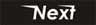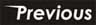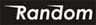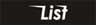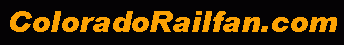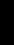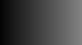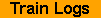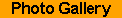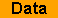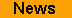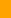Photo By: Kevin Morgan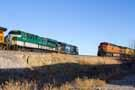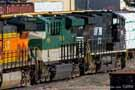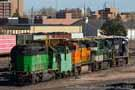Here is an obligatory roster shot of Norfolk Southern's "Southern" Heritage Unit. I think this is one of the sharper looking heritage units that Norfolk Southern has. Note the "K" on the cab behind "8099". This is part of a number check system that Southern used.

To find the letter, do the following:
1st digit + (2nd digit times 2) + 3rd digit + (4th digit times 2). If doubling a digit results in a two digit number (9 x 2 = 18), then add the two digits together to get a single digit. So 9 x 2 = 18, 1 + 8 = 9.

So, using this formula, (8 + 0 + 9 + ((9x2=18), (1+8=9), so 9)) = 26. Subtract that number from the next even multiple of 10. So 30 - 26 = 4. Then, find the letter that corresponds to the calculated number:
0 = A,
1 = F,
2 = H,
2 = J,
4 = K,
5 = L,
6 = R,
7 = T,
8 = W,
9 = X

From the calculation above, the single digit number we calculated was "4", and "4" matches "K", which is exactly the letter that is on the cab!
Email Questions or Comments to the Webmaster.

 1323 views
 Thursday, December 11, 2014 at 2:55:54 PM image date

 Location Information City or County and State Broomfield, CO Subdivision Front Range (BNSF) Milepost or Control Point Broomfield

 Train Information
 Railroad Norfolk Southern Model ES44AC Unit Number NS 8099

 Symbol HLAUDEN

 Photo Information
 Camera Make/Model Canon / Canon EOS 7D ISO 320 Flash Did Not Fire

 Focal Length 31 mm Shutter 1/500 seconds Aperture f/10Check out ColoradoRailfan.com on Facebook

 The D&RGW Site Ring This site owned by: Kevin Morgan Add Your Site